Next: K-means Up: Flat clustering Previous: Cardinality - the number   Contents   Index

Evaluation of clustering

Typical objective functions in clustering formalize the goal of attaining high intra-cluster similarity (documents within a cluster are similar) and low inter-cluster similarity (documents from different clusters are dissimilar). This is an internal criterion for the quality of a clustering. But good scores on an internal criterion do not necessarily translate into good effectiveness in an application. An alternative to internal criteria is direct evaluation in the application of interest. For search result clustering, we may want to measure the time it takes users to find an answer with different clustering algorithms. This is the most direct evaluation, but it is expensive, especially if large user studies are necessary.

As a surrogate for user judgments, we can use a set of classes in an evaluation benchmark or gold standard (see Section 8.5 , page 8.5 , and Section 13.6 , page 13.6 ). The gold standard is ideally produced by human judges with a good level of inter-judge agreement (see Chapter 8 , page 8.1 ). We can then compute an external criterion that evaluates how well the clustering matches the gold standard classes. For example, we may want to say that the optimal clustering of the search results for jaguar in Figure 16.2 consists of three classes corresponding to the three senses car, animal, and operating system. In this type of evaluation, we only use the partition provided by the gold standard, not the class labels.

This section introduces four external criteria of clustering quality. Purity is a simple and transparent evaluation measure. Normalized mutual information can be information-theoretically interpreted. The Rand index penalizes both false positive and false negative decisions during clustering. The F measure in addition supports differential weighting of these two types of errors.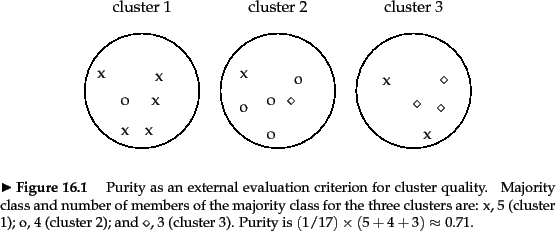To compute purity , each cluster is assigned to the class which is most frequent in the cluster, and then the accuracy of this assignment is measured by counting the number of correctly assigned documents and dividing by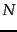. Formally: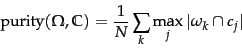(182)

where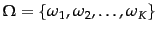is the set of clusters and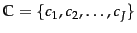is the set of classes. We interpret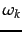as the set of documents inand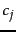as the set of documents inin Equation 182.

We present an example of how to compute purity in Figure 16.4 .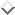Bad clusterings have purity values close to 0, a perfect clustering has a purity of 1 . Purity is compared with the other three measures discussed in this chapter in Table 16.2 .

Table 16.2: The four external evaluation measures applied to the clustering in Figure 16.4 .
 purity NMI RI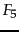lower bound 0.0 0.0 0.0 0.0 maximum 1 1 1 1 value for Figure 16.4 0.71 0.36 0.68 0.46

High purity is easy to achieve when the number of clusters is large - in particular, purity is 1 if each document gets its own cluster. Thus, we cannot use purity to trade off the quality of the clustering against the number of clusters.

A measure that allows us to make this tradeoff is normalized mutual information or NMI :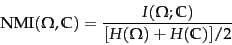(183)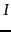is mutual information (cf. Chapter 13 , page 13.5.1 ):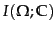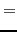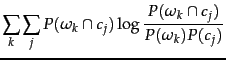(184)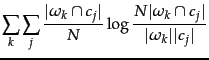(185)

where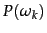,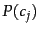, and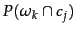are the probabilities of a document being in cluster, class, and in the intersection ofand, respectively. Equation 185 is equivalent to Equation 184 for maximum likelihood estimates of the probabilities (i.e., the estimate of each probability is the corresponding relative frequency).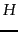is entropy as defined in Chapter 5 (page 5.3.2 ):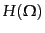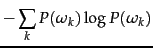(186)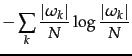(187)

where, again, the second equation is based on maximum likelihood estimates of the probabilities.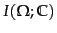in Equation 184 measures the amount of information by which our knowledge about the classes increases when we are told what the clusters are. The minimum ofis 0 if the clustering is random with respect to class membership. In that case, knowing that a document is in a particular cluster does not give us any new information about what its class might be. Maximum mutual information is reached for a clustering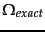that perfectly recreates the classes - but also if clusters inare further subdivided into smaller clusters (Exercise 16.7 ). In particular, a clustering with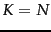one-document clusters has maximum MI. So MI has the same problem as purity: it does not penalize large cardinalities and thus does not formalize our bias that, other things being equal, fewer clusters are better.

The normalization by the denominator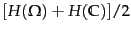in Equation 183 fixes this problem since entropy tends to increase with the number of clusters. For example,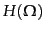reaches its maximum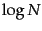for, which

ensures that NMI is low for. Because NMI is normalized, we can use it to compare clusterings with different numbers of clusters. The particular form of the denominator is chosen becauseis a tight upper bound on(Exercise 16.7 ). Thus, NMI is always a number between 0 and 1.

An alternative to this information-theoretic interpretation of clustering is to view it as a series of decisions, one for each of the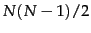pairs of documents in the collection. We want to assign two documents to the same cluster if and only if they are similar. A true positive (TP) decision assigns two similar documents to the same cluster, a true negative (TN) decision assigns two dissimilar documents to different clusters. There are two types of errors we can commit. A (FP) decision assigns two dissimilar documents to the same cluster. A (FN) decision assigns two similar documents to different clusters. The Rand index ( ) measures the percentage of decisions that are correct. That is, it is simply accuracy (Section 8.3 , page 8.3 ).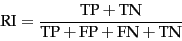As an example, we compute RI for Figure 16.4 . We first compute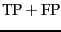. The three clusters contain 6, 6, and 5 points, respectively, so the total number of positives'' or pairs of documents that are in the same cluster is: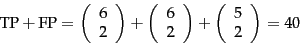(188)

Of these, the x pairs in cluster 1, the o pairs in cluster 2, the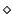pairs in cluster 3, and the x pair in cluster 3 are true positives: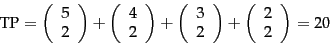(189)

Thus,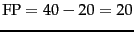.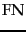and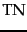are computed similarly, resulting in the following contingency table:

 Same cluster Different clusters Same class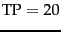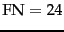Different classes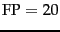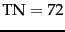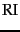is then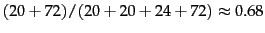.

The Rand index gives equal weight to false positives and false negatives. Separating similar documents is sometimes worse than putting pairs of dissimilar documents in the same cluster. We can use the F measure measuresperf to penalize false negatives more strongly than false positives by selecting a value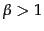, thus giving more weight to recall.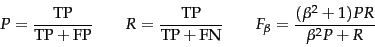Based on the numbers in the contingency table,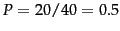and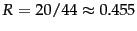. This gives us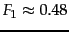for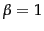and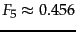for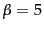. In information retrieval, evaluating clustering with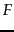has the advantage that the measure is already familiar to the research community.

Exercises.

• Replace every point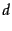in Figure 16.4 with two identical copies ofin the same class. (i) Is it less difficult, equally difficult or more difficult to cluster this set of 34 points as opposed to the 17 points in Figure 16.4 ? (ii) Compute purity, NMI, RI, andfor the clustering with 34 points. Which measures increase and which stay the same after doubling the number of points? (iii) Given your assessment in (i) and the results in (ii), which measures are best suited to compare the quality of the two clusterings?Next: K-means Up: Flat clustering Previous: Cardinality - the number   Contents   Index